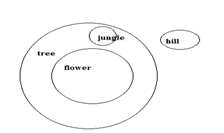# SSC CGL 2018 Practice Test Papers | Reasoning Ability (Day-2)

Dear Aspirants, Here we have given the Important SSC CGL Exam 2018 Practice Test Papers. Candidates those who are preparing for SSC CGL 2018 can practice these questions to get more confidence to Crack SSC CGL 2018 Examination.

[WpProQuiz 2610]

Click “Start Quiz” to attend these Questions and view Explanation

1. If 3rd March 2016 was Thursday then what will be the day of December 3 2016?
1. Tuesday
2. Sunday
3. Thursday
4. Saturday
1. A word is represented by only one set of numbers as given in any one of the alternatives. The set of numbers is given in any one of the alternatives. The sets of numbers given in the alternatives are represented by two classes of alphabets as in two matrices given below. The columns and rows of matrix I are numbered from 0 to 4 And that matrix II are numbered from 5 to 9. A letter from these matrices can be represented first by its row and next by its column. Ex: A can be represented by. Similarly you have to identify the set for the word ‘STUDY’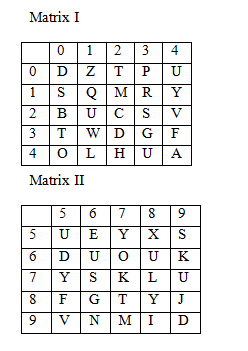a.76,86,79,65,22

b.10,87,66,99,14

c.85,87,79,96,33

d.40,87,66,33,22

1. Introducing a boy Sheela says he is the son of my Father’s daughter. How Sheela is related to that boy?
1. Sister
2. Mother
3. Aunt
4. Either b or c
1. Choose a figure which would most closely resemble the unfolded form of Figure (Z).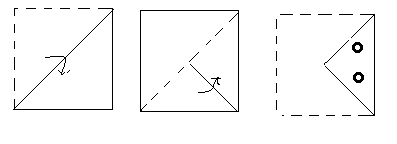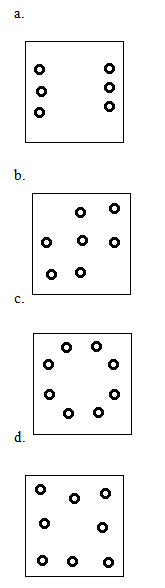1. If a mirror is placed on the line AB then which of the following is right image of the figure.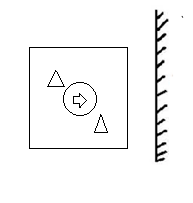1. Identify the diagram that best represents the relationship between the given classes.

Living thing, Reptile, Lizard, Table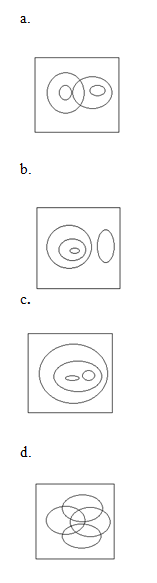1. A series is given with one term missing. Choose the correct alternative from the given ones that will  complete the series.

AF, GM, NU,??

1. XC
2. VD
3. WD
4. D.YE
1. Statement: All flowers are tree. No jungle is hill. All jungle are tree.

Conclusion:

A) some flowers are not hill.

B) No tree is hill.

C) Some jungles are flowers.

1. None follows
2. A and B follows
3. A and C follows
4. B and C follows
1. Arrange the given words in the sequence in which they occur in the dictionary.

i.Exalt

ii.Exit

iii. Example

iv .Exotic

1. iv, ii, i, iii
2. iii, iv, ii, i
3. iv, iii, i, ii
4. i, iii ,ii, iv
1. If A denotes ‘added to’ B denotes ‘multiplied by’, C denotes ‘divided by’ D denotes ‘subtracted from’ then what is the value of the given expression?

40 D 81 C 27 A 10 B 3=?

a.67

b.45

c.39

d.65

Explanation:

No. of odd days from March 3 to December 3=3+2+3+2+3+3+2+3+2=23

No. of odd days in 23=23%7 = 2 [%- Remainder of 23/7=2]

Thursday +2 = Saturday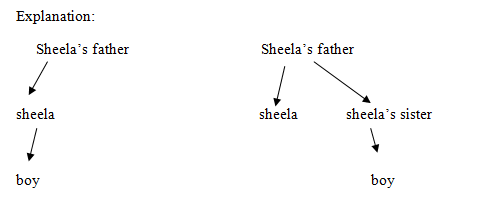Explanation:

AF = 6 – 1 = 5

GM = 13 – 7 = 6

NU = 21 – 14 = 7

VD = 30 – 22 = 8